# MA.912.DP.3.3Export Print
Given a two-way relative frequency table or segmented bar graph summarizing categorical bivariate data, interpret joint, marginal and conditional relative frequencies in terms of a real-world context.

### Examples

Algebra 1 Example: Given the relative frequency table below, the ratio of true positives to false positives can be determined as 7.2 to 4.55, which is about 3 to 2, meaning that a randomly selected person who tests positive for diabetes is about 50% more likely to have diabetes than not have it.

 Positive Negative Total Has diabetes 7.2% 1.8% 9% Doesn't have diabetes 4.55% 86.45% 91%

### Clarifications

Clarification 1: Instruction includes problems involving false positive and false negatives.
General Information
Subject Area: Mathematics (B.E.S.T.)
Strand: Data Analysis and Probability
Status: State Board Approved

## Benchmark Instructional Guide

### Terms from the K-12 Glossary

• Categorical Data

### Vertical Alignment

Previous Benchmarks

Next Benchmarks

### Purpose and Instructional Strategies

In grades 7 and 8, students explored the relationship between experimental and theoretical probabilities. In Algebra I, students study associations in bivariate categorical data using conditional relative frequencies and they apply these to real-world contexts. In later courses, students will study experimental and theoretical conditional probabilities in real-world contexts.
• In this benchmark, students will calculate and interpret joint, marginal and conditional relative frequencies in terms of a real-world context.
• When medical studies are involved the following terminology is used.
• True Positive (TP) means that a person who has a disease is correctly identified as having that disease by the test.
• False Positive (FP) means that a person who does not have a disease is incorrectly identified as having that disease by the test.
• True Negative (TN) means that a person who does not have a disease is correctly identified as not having that disease by the test.
• False Negative (FN) means that a person who has a disease is incorrectly identified as not having that disease by the test.
• In order to interpret the joint, marginal and conditional relative frequencies, students must know the difference between them.
• Marginal relative frequencies Guide students to understand that the total column and total row are in the “margins” of the table, thus they are referred to as the marginal relative frequencies.
• Joint relative frequencies Guide students to connect that the word joint refers to the coming together of more than one, therefore the term joint relative frequency refers to combination of two categories or conditions happening together.
• Conditional relative frequencies Guide students to connect that the word conditional refers imposing a constraint on one of the two variables, therefore the term conditional relative frequency refers to the percentage in a category for one variable when one has put a constraint on the other variable.
• For example, the relative frequency table below describes whether a student completed a test review sheet or not and whether they passed the test. To determine the conditional relative frequency of those who passed the test given that they completed the review, one could take the ratio between the joint relative frequency for passing the test in the first row and the marginal relative frequency in the first row, which is $\frac{\text{54%}}{\text{60%}}$ which is equivalent to 90%. One could say that 90% of those who completed the review passed the test.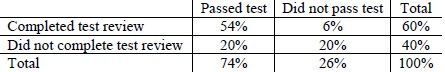### Common Misconceptions or Errors

• Students may not understand how the two-way relative frequency table connects with true positives/false positives and false negatives/true negatives.
• Students may not understand how to create the proper ratio for a given purpose.
• Students may not understand how to connect the ratio to the context.

### Strategies to Support Tiered Instruction

• Teacher co-creates a graphic organizer to distinguish between the positives and negatives.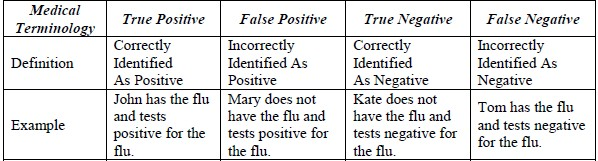• Teacher models distinguishing between relative frequencies given basic equations.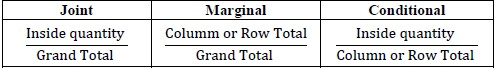• Teacher provides opportunity to highlight the middle of the two-way table to distinguish the conditional and joint relative frequencies from marginal relative frequencies. The teacher models a joint, conditional or marginal relative frequency given the instructional task/problem.
• For example, when determining the conditional relative frequency that given that a student is an 8th grader, that student studies on the weekend, teacher can highlight the numbers in the two-way table below. Then, students can determine the relative frequency to be 51.5% since $\frac{\text{170}}{\text{330}}$ = 0.5151.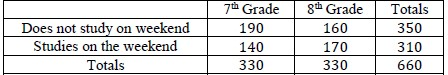• Teacher co-creates a graphic organizer for each of the types of relative frequencies (joint, marginal and conditional) and highlights key similarities and differences.

• The relative frequency table below describes the members of the local sports club, showing whether they are male or female and whether they play disc golf or not.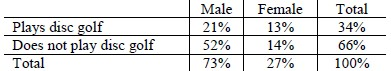• Part A. Compare the conditional relative frequency of being male given that they play disc golf and being female given that they play disc golf.
• Part B. Is there an association between being female and playing disc golf?
• Part C. Determine a possible reason for the association found in Part B. How do you think this may compare with any association that might exist in the general population between being female and playing disc golf?

### Instructional Items

Instructional Item 1
• In 2016, a clinical study in a small town of New Hampshire found that about 13% of its population had the flu and 87% did not. The study also showed that 19% of the people who had the flu tested negative while 8% of the people who did not have the flu tested positive. The relative frequency table below summarizes the data.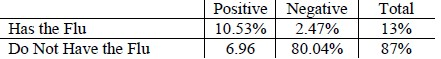Determine the ratio of true positives to false positives and interpret that in terms of the context.

*The strategies, tasks and items included in the B1G-M are examples and should not be considered comprehensive.

## Related Courses

This benchmark is part of these courses.
1200320: Algebra 1 Honors (Specifically in versions: 2014 - 2015, 2015 - 2022, 2022 and beyond (current))
1200400: Foundational Skills in Mathematics 9-12 (Specifically in versions: 2014 - 2015, 2015 - 2022, 2022 and beyond (current))
1210300: Probability and Statistics Honors (Specifically in versions: 2014 - 2015, 2015 - 2019, 2019 - 2022, 2022 and beyond (current))
1200388: Mathematics for Data and Financial Literacy Honors (Specifically in versions: 2022 and beyond (current))
1200384: Mathematics for Data and Financial Literacy (Specifically in versions: 2022 and beyond (current))
7912120: Access Mathematics for Data and Financial Literacy (Specifically in versions: 2022 - 2023, 2023 and beyond (current))

## Related Access Points

Alternate version of this benchmark for students with significant cognitive disabilities.
MA.912.DP.3.AP.3: Given a segmented bar graph summarizing categorical bivariate data, select the interpretation in terms of a real-world context.

## Related Resources

Vetted resources educators can use to teach the concepts and skills in this benchmark.

## Formative Assessments

School Start Time:

Students are asked to describe an association between two variables given a table of relative frequencies by column.

Type: Formative Assessment

Conditional Relative Frequency:

Students are asked to use a two-way frequency table to interpret two different conditional relative frequencies.

Type: Formative Assessment

Siblings and Pets:

Students are asked to describe an association between two variables given a table of relative frequencies by row.

Type: Formative Assessment

## Original Student Tutorial

Data and Frequencies:

Learn to define, calculate, and interpret marginal frequencies, joint frequencies, and conditional frequencies in the context of the data with this interactive tutorial.

Type: Original Student Tutorial

## MFAS Formative Assessments

Conditional Relative Frequency:

Students are asked to use a two-way frequency table to interpret two different conditional relative frequencies.

School Start Time:

Students are asked to describe an association between two variables given a table of relative frequencies by column.

Siblings and Pets:

Students are asked to describe an association between two variables given a table of relative frequencies by row.

## Original Student Tutorials Mathematics - Grades 9-12

Data and Frequencies:

Learn to define, calculate, and interpret marginal frequencies, joint frequencies, and conditional frequencies in the context of the data with this interactive tutorial.

## Student Resources

Vetted resources students can use to learn the concepts and skills in this benchmark.

## Original Student Tutorial

Data and Frequencies:

Learn to define, calculate, and interpret marginal frequencies, joint frequencies, and conditional frequencies in the context of the data with this interactive tutorial.

Type: Original Student Tutorial

## Parent Resources

Vetted resources caregivers can use to help students learn the concepts and skills in this benchmark.